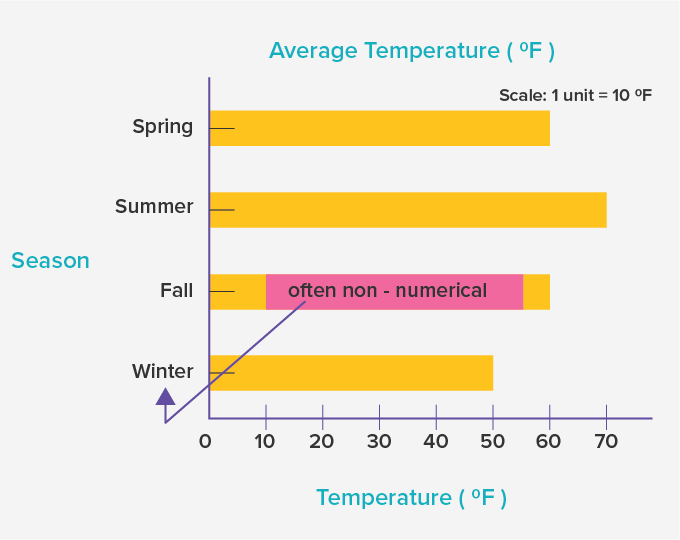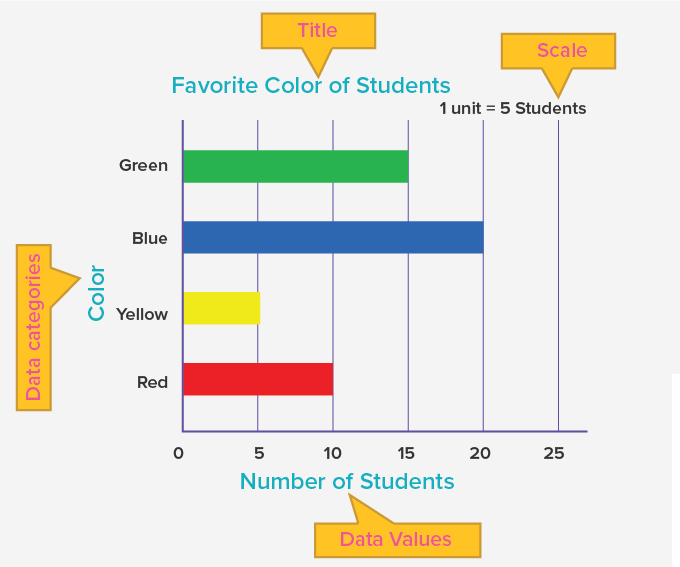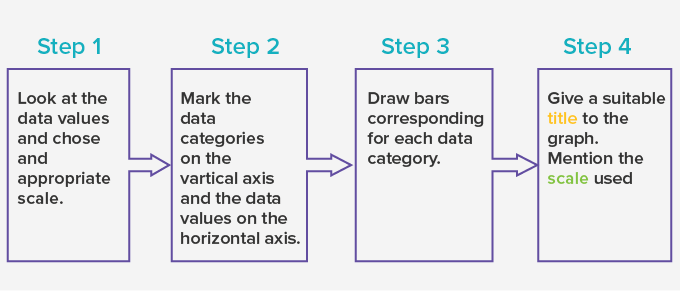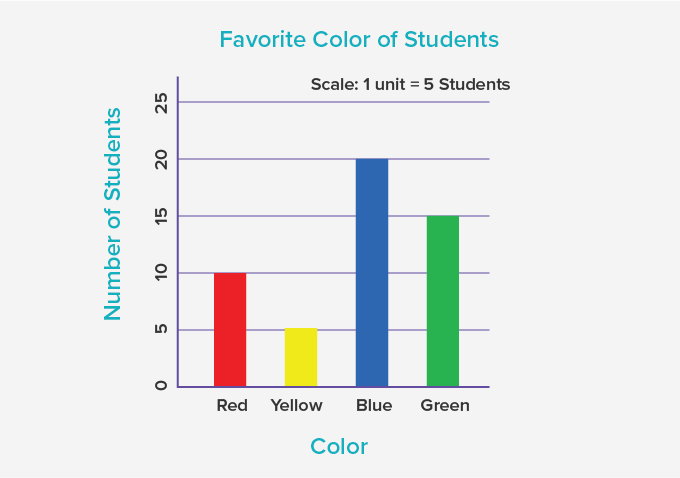# Horizontal Bar Graph - Definition with Examples

The Complete K-5 Math Learning Program Built for Your Child

• 40 Million Kids

Loved by kids and parent worldwide

• 50,000 Schools

Trusted by teachers across schools

• Comprehensive Curriculum

Aligned to Common Core

## Horizontal Bar Graph

Bar Graph

A bar graph is a graph with rectangular bars with lengths and heights proportional to the values that they represent.

• On one axis of the graph, it shows the data categories that are being compared.

• The other axis represents the values corresponding to each data category.

Horizontal bar graph

Horizontal bar graphs represent the data horizontally. It is a graph whose bars are drawn horizontally. The data categories are shown on the vertical axis and the data values are shown on the horizontal axis. The length of each bar is equal to the value corresponding the data category and all bars go across from left to right.## Reading a horizontal bar graph

• The title of the horizontal bar graph tells about the data being represented by the graph.

• The vertical axis represents the data categories. Here, the data categories are the colors.

• The horizontal axis represents the values corresponding to each data value. Here, the data values are the number of students who like a particular color represented on the vertical axis.

• The scale shows the value of 1 unit on the horizontal axis.From the given horizontal bar graph, we can infer that on the  scale1unit represents 5 students and 10 students like the red, 5 like yellow, 20 like blue and 15 like green.

## Drawing a horizontal bar graph

Here, is the data showing the number of children who visited a library during the first three months of a year.

 Children in a Library Month Number of children January 40 February 35 March 50

To represent this data on a horizontal bar graph:So, taking a scale of 10, we get the horizontal bar graph as:

 Fun Facts The other type of bar graph is the vertical bar graph, wherein the data is represented through vertical bars. The x-axis and the y-axis are switched in a vertical bar graph.Won Numerous Awards & Honors